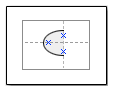# Check Pole-Zero Characteristics

Check that bounds on pole locations are satisfied during simulation

## Library

•## Description

This block is the same as the Pole-Zero Plot block except for different default parameter settings in the Bounds tab.

Check that approximate second-order bounds on the pole locations of a linear system, computed from a nonlinear Simulink model, are satisfied during simulation.

The Simulink model can be continuous-time, discrete-time or multirate and can have time delays. Because you can specify only one linearization input/output pair in this block, the linear system is single-input single-output (SISO).

During simulation, the software linearizes the portion of the model between specified linearization inputs and outputs, computes the poles and zeros, and checks that the poles satisfy the specified bounds:

• If all bounds are satisfied, the block does nothing.

• If a bound is not satisfied, the block asserts and a warning message appears in the MATLAB® Command Window. You can also specify that the block:

• Evaluate a MATLAB expression.

• Stop the simulation and bring that block into focus.

During simulation, the block can also output a logical assertion signal.

• If all bounds are satisfied, the signal is true (`1`).

• If any bound is not satisfied, the signal is false (`0`).

You can add multiple Check Pole-Zero Characteristics blocks in your model to check approximate second-order bounds on various portions of the model.

You can also plot the poles and zeros on a pole-zero map and graphically verify that the poles satisfy the bounds.

This block and the other Model Verification blocks test that the linearized behavior of a nonlinear Simulink model is within specified bounds during simulation.

• When a model does not violate any bound, you can disable the block by clearing the assertion option. If you modify the model, you can re-enable assertion to ensure that your changes do not cause the model to violate a bound.

• When a model violates any bound, you can use Simulink Design Optimization™ software to optimize the linear system to meet the specified requirements in this block.

You can save the linear system as a variable in the MATLAB workspace.

The block does not support code generation and can be used only in `Normal` simulation mode.

## Parameters

The following table summarizes the Pole-Zero Plot block parameters, accessible via the block parameter dialog box. For more information, see Parameters in the Pole-Zero Plot block reference page.

Configure linearization.Specify inputs and outputs (I/Os).

In Linearizations tab:

• Linearization inputs/outputs

• Click a model signal to add it as a linearization I/O

Specify settings.

In Linearizations tab:

• Linearize on

• Snapshot times

• Trigger type

Specify algorithm options.

In Linearizations tab:

• Enable zero-crossing detection

• Use exact delays

• Linear system sample time

• Sample time rate conversion method

Specify labels for linear system I/Os and state names.

In Linearizations tab:

• Use full block names

• Use bus signal names

Specify bounds on the linear system for assertion.

In Bounds tab:

• Include settling time bound in assertion

• Include percent overshoot bound in assertion

• Include damping ratio bound in assertion

• Include natural frequency bound in assertion

Specify assertion options (only when you specify bounds on the linear system).

In Assertion tab:

• Enable assertion

• Simulation callback when assertion fails (optional)

• Stop simulation when assertion fails

• Output assertion signal

Save linear system to MATLAB workspace. Save data to workspace in Logging tab.
View bounds violations graphically in a plot window.
Display plot window instead of block parameters dialog box on double-clicking the block.Show plot on block open

Pole-Zero Plot

Introduced in R2010b